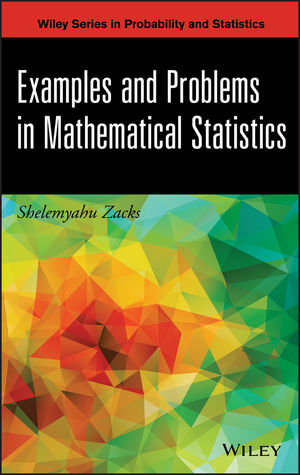## ABC by classification

Posted in pictures, Statistics, Travel, University life with tags , , , , , , , , , , on December 21, 2021 by xi'anAs a(nother) coincidence, yesterday, we had a reading group discussion at Paris Dauphine a few days after Veronika Rockova presented the paper in person in Oaxaca. The idea in ABC by classification that she co-authored with Yuexi Wang and Tetsuya Kaj is to use the empirical Kullback-Leibler divergence as a substitute to the intractable likelihood at the parameter value θ. In the generalised Bayes setting of Bissiri et al. Since this quantity is not available it is estimated as well. By a classification method that somehow relates to Geyer’s 1994 inverse logistic proposal, using the (ABC) pseudo-data generated from the model associated with θ. The convergence of the algorithm obviously depends on the choice of the discriminator used in practice. The paper also makes a connection with GANs as a potential alternative for the generalised Bayes representation. It mostly focus on the frequentist validation of the ABC posterior, in the sense of exhibiting a posterior concentration rate in n, the sample size, while requiring performances of the discriminators that may prove hard to check in practice. Expanding our 2018 result to this setting, with the tolerance decreasing more slowly than the Kullback-Leibler estimation error.

Besides the shared appreciation that working with the Kullback-Leibler divergence was a nice and under-appreciated direction, one point that came out of our discussion is that using the (estimated) Kullback-Leibler divergence as a form of distance (attached with a tolerance) is less prone to variability (or more robust) than using directly (and without tolerance) the estimate as a substitute to the intractable likelihood, if we interpreted the discrepancy in Figure 3 properly. Another item was about the discriminator function itself: while a machine learning methodology such as neural networks could be used, albeit with unclear theoretical guarantees, it was unclear to us whether or not a new discriminator needed be constructed for each value of the parameter θ. Even when the simulations are run by a deterministic transform.

## minimaxity of a Bayes estimator

Posted in Books, Kids, Statistics, University life with tags , , , , , on February 2, 2015 by xi'anToday, while in Warwick, I spotted on Cross Validated a question involving “minimax” in the title and hence could not help but look at it! The way I first understood the question (and immediately replied to it) was to check whether or not the standard Normal average—reduced to the single Normal observation by sufficiency considerations—is a minimax estimator of the normal mean under an interval zero-one loss defined by$\mathcal{L}(\mu,\hat{\mu})=\mathbb{I}_{|\mu-\hat\mu|>L}=\begin{cases}1 &\text{if }|\mu-\hat\mu|>L\\ 0&\text{if }|\mu-\hat{\mu}|\le L\\ \end{cases}$

where L is a positive tolerance bound. I had not seen this problem before, even though it sounds quite standard. In this setting, the identity estimator, i.e., the normal observation x, is indeed minimax as (a) it is a generalised Bayes estimator—Bayes estimators under this loss are given by the centre of an equal posterior interval—for this loss function under the constant prior and (b) it can be shown to be a limit of proper Bayes estimators and its Bayes risk is also the limit of the corresponding Bayes risks. (This is a most traditional way of establishing minimaxity for a generalised Bayes estimator.) However, this was not the question asked on the forum, as the book by Zacks it referred to stated that the standard Normal average maximised the minimal coverage, which amounts to the maximal risk under the above loss. With the strange inversion of parameter and estimator in the minimax risk:$\sup_\mu\inf_{\hat\mu} R(\mu,\hat{\mu})\text{ instead of } \sup_\mu\inf_{\hat\mu} R(\mu,\hat{\mu})$

which makes the first bound equal to 0 by equating estimator and mean μ. Note however that I cannot access the whole book and hence may miss some restriction or other subtlety that would explain for this unusual definition. (As an aside, note that Cross Validated has a protection against serial upvoting, So voting up or down at once a large chunk of my answers on that site does not impact my “reputation”!)